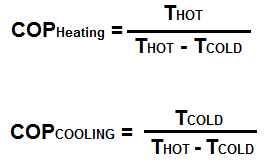﻿ Coefficient of Performance Calculator ﻿# Coefficient of Performance CalculatorEnter TCOLD Kelvin Celsius Fahrenheit Enter the THOT Kelvin Celsius Fahrenheit Calculating Cooling Heating

Coefficient of Performance:

This coefficient of performance (COP) calculator calculates the ratio of heating or cooling provided to work required.

The most basic formula to COP is Q/W, where Q is the heat supplied to or removed from the reservoir and W is the work done by the heat pump.

Therefore, you can see based on the formula that higher COPs provide better system performance and normally equates to lower operating costs.

If you take formulas in thermodynamics, you can get that COPHeating= THOT/(THOT - TCOLD). Similarly, you can get that COPCOOLING= TCOLD/(THOT - TCOLD).

And these formulas above are what this calculator uses to calculate the coefficient of performance of a heat pump.

When calculating the coefficient of performance, an absolute temperature unit must be used such as Kelvin. This calculator uses Kelvin as the base unit to make all calculations. If you choose Celsius or Fahrenheit, they are converted to Kelvin; and then the calculations are done based on Kelvin.

In order to convert Celsius to Kelvin, 273.15 is added to the Celsius value.

In order to convert Fahrenheit to Kelvin, the formula to do is, TemperatureKELVIN= (T(°F) + 459.67)× 5/9.

### Example

Calculate coefficient of performance (COP) of a heating system in which the TCOLD value is 10K and the THOT value is 200K.

COPHEATING= THOT/(THOT - TCOLD)= 200/(200-10)= 1.05.

So the COP is 1.05 in this case.

Related Resources

﻿